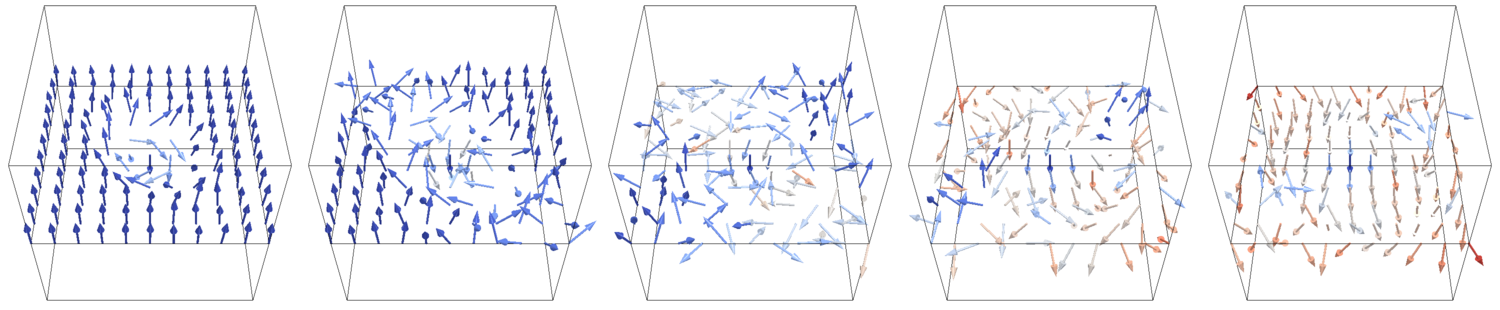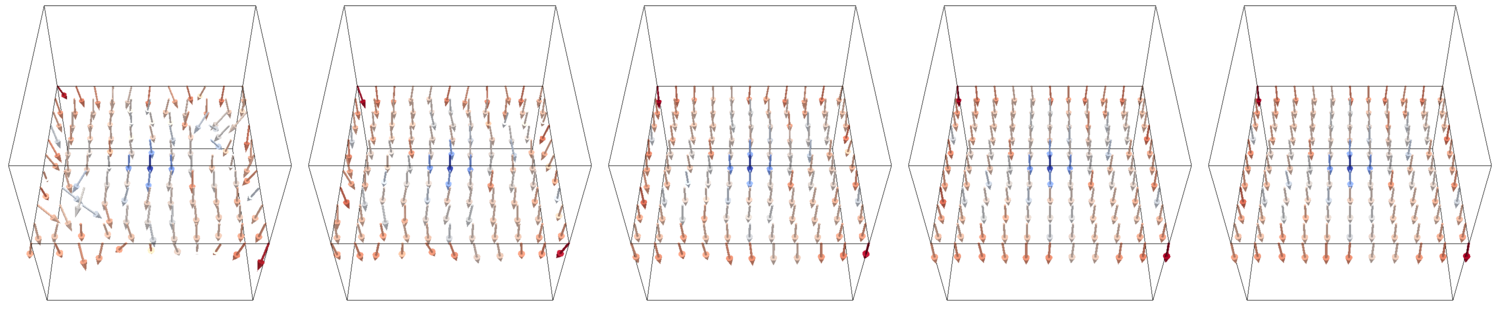# Associated Project 4 • Numerics and foundations of computational micro-magnetism (11/2017 - 8/2019)Figure 1. Typical dynamics of magnetization in the presence of an external field.

Our group addresses problems in computational and numerical mathematics with great practical relevance. The different applications range from magnetic storage devices and magnetic sensors to embedded systems and they all have in common that they can be mathematically described by the Landau–Lifshitz–Gilbert (LLG) equation coupled with the Maxwell equations.

However, even the standard techniques for solving those equations on a deterministic level still lack the theoretical underpinning, which one would expect from something used so widely in industry and science. Only very recently, first error estimates for a popular algorithm for the deterministic LLG–Maxwell equations have been proved. However, in view of the applications, those deterministic simulations are not enough, as random thermal fluctuations become more significant as the devices shrink in size.

The Landau–Lifshitz–Gilbert (LLG) equation is a quasi-linear parabolic PDE of the form \begin{alignat*}{2} \partial_t\boldsymbol{m} - \alpha\boldsymbol{m}\times\partial_t\boldsymbol{m} &= -\boldsymbol{m} \times \boldsymbol{H}_{\rm eff}\quad &&\text{in }[0,T]\times D,\\ \partial_{\boldsymbol{n}}\boldsymbol{m} &=\boldsymbol{0}\quad &&\text{on }[0,T]\times\partial D,\\ \boldsymbol{m}(0,\cdot) &= \boldsymbol{m}^0\quad &&\text{in }D, \end{alignat*} where the magnetization $\boldsymbol{m}$ satisfies $|\boldsymbol{m}(t,\cdot)|=1$ for all $t\in[0,T]$ and $\boldsymbol{H}_{\rm eff} =\Delta \boldsymbol{m} + \text{lower order terms}$. Here $D\subseteq \mathbb{R}^3$ is the magnetic domain. Physicists are often interested in the coupling of this equation to the Maxwell-system posed on the whole of $\mathbb{R}^3$ \begin{align*} \nabla\times \boldsymbol{H} &=\partial_t\boldsymbol{E} +\sigma \boldsymbol{E},\\ \nabla\times \boldsymbol{E} &=-\mu_0\partial_t(\boldsymbol{H}+\boldsymbol{m}),\\ \operatorname{div}(\boldsymbol{H}+\boldsymbol{m})&=0,\\ \operatorname{div}(\varepsilon\boldsymbol{E})&=0. \end{align*}

This coupled system is widely used in the simulation of magnetic storage devices, permanent magnets, and spintronic devices. Since any useful discretization of the system above will result in a huge (non-)linear system of equations (three components of $\boldsymbol{m}$, $\boldsymbol{E}$, and $\boldsymbol{H}$ in each discretization point in $\mathbb{R}^3$), it is essential to develop fast numerical algorithms for this task.

## Goals

• Finite-Element/Boundary-Element coupling with convolution quadrature for the discretization of the Maxwell system.
• Developement of adaptive algorithms and reliable error estimators.
• Efficient treatment of stochastic noise with fast sampling and multi-level/multi-index techniques.

## Publications

1. and . Exponential convergence in $H^1$ of $hp$-FEM for Gevrey regularity with isotropic singularities uncertainty quantification. Numer. Math., 1–24, November . Online first. [preprint] [bibtex]

## Preprints

1. , , and . Improved efficiency of a multi-index FEM for computational uncertainty quantification. CRC 1173 Preprint 2018/22, Karlsruhe Institute of Technology, August . [bibtex]

2. and . Sparse compression of expected solution operators. CRC 1173 Preprint 2018/21, Karlsruhe Institute of Technology, August . [bibtex]

Project-specific staff
NamePhoneE-Mail+49 721 608-43036 jan bohnBuv4∂kit edu
+43 58801-10154 michael feischlVug5∂tuwien ac at